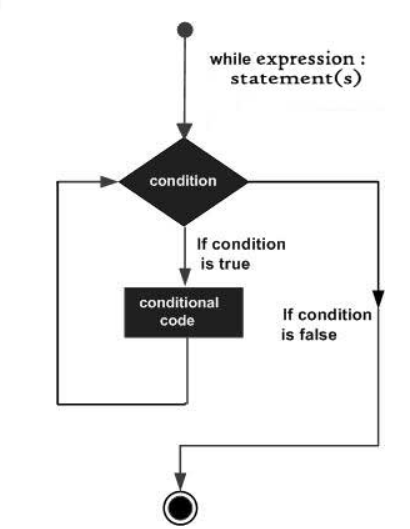×

Search anything:

# Learn about While loop in Python

#### Software Engineering Python while loopGet this book -> Problems on Array: For Interviews and Competitive Programming

Python programming language provides various types of loops to handle looping requirements. A while loop statement in Python programming language repeatedly executes a target statement as long as a given condition is true. When the condition becomes false, the line immediately after the loop in program is executed.

## Syntax

``````   while expression:
statement(s)
``````

Here, statement(s) may be a single statement or a block of statements. The condition may be any expression, and true is any non-zero value. The loop iterates while the condition is true.

When the condition becomes false, program control passes to the line immediately following the loop.

In Python, all the statements indented by the same number of character spaces after a programming construct are considered to be part of a single block of code. Python uses indentation as its method of grouping statements.

## FlowchartHere, key point of the while loop is that the loop might not ever run. When the condition is tested and the result is false, the loop body will be skipped and the first statement after the while loop will be executed.

## Example

``````   count = 0
while (count < 3):
count = count + 1
print("Hello Geek")
``````

## The Infinite while Loop in Python

A loop becomes infinite loop if a condition never becomes FALSE. You must use caution when using while loops because of the possibility that this condition never resolves to a FALSE value. This results in a loop that never ends. Such a loop is called an infinite loop.

An infinite loop might be useful in client/server programming where the server needs to run continuously so that client programs can communicate with it as and when required.

## Example

``````var = 1
while var == 1 :  # This constructs an infinite loop
num = raw_input("Enter a number  :")
print "You entered: ", num

print "Good bye!"
``````

Above example goes in an infinite loop and you need to use CTRL+C to exit the program.

## Using else Statement with Loops

Python supports to have an else statement associated with a loop statement.

• If the else statement is used with a for loop, the else statement is executed when the loop has exhausted iterating the list.

• If the else statement is used with a while loop, the else statement is executed when the condition becomes false.

The following example illustrates the combination of an else statement with a while statement that prints a number as long as it is less than 5, otherwise else statement gets executed.

## Example

``````count = 0
while count < 5:
print count, " is  less than 5"
count = count + 1
else:
print count, " is not less than 5"
``````

## Single Statement While loops

Similar to the if statement syntax, if your while clause consists only of a single statement, it may be placed on the same line as the while header.

Here is the syntax and example of a one-line while clause:

## Example

``````flag = 1
while (flag): print 'Given flag is really true!'
print "Good bye!"
``````

Note: It is suggested not to use this type of loops as it is a never ending infinite loop where the condition is always true and you have to forcefully terminate the compiler.